# Heat map in R

## The `heatmap` function

The `heatmap` function can draw a heat map in R from a matrix. In the following examples we are going to use a square matrix but note that the number of rows and columns doesn’t need to be the same.

``````# Matrix
m <- matrix(rnorm(100), ncol = 10)
colnames(m) <- paste("Col", 1:10)
rownames(m) <- paste("Row", 1:10)

heatmap(m)``````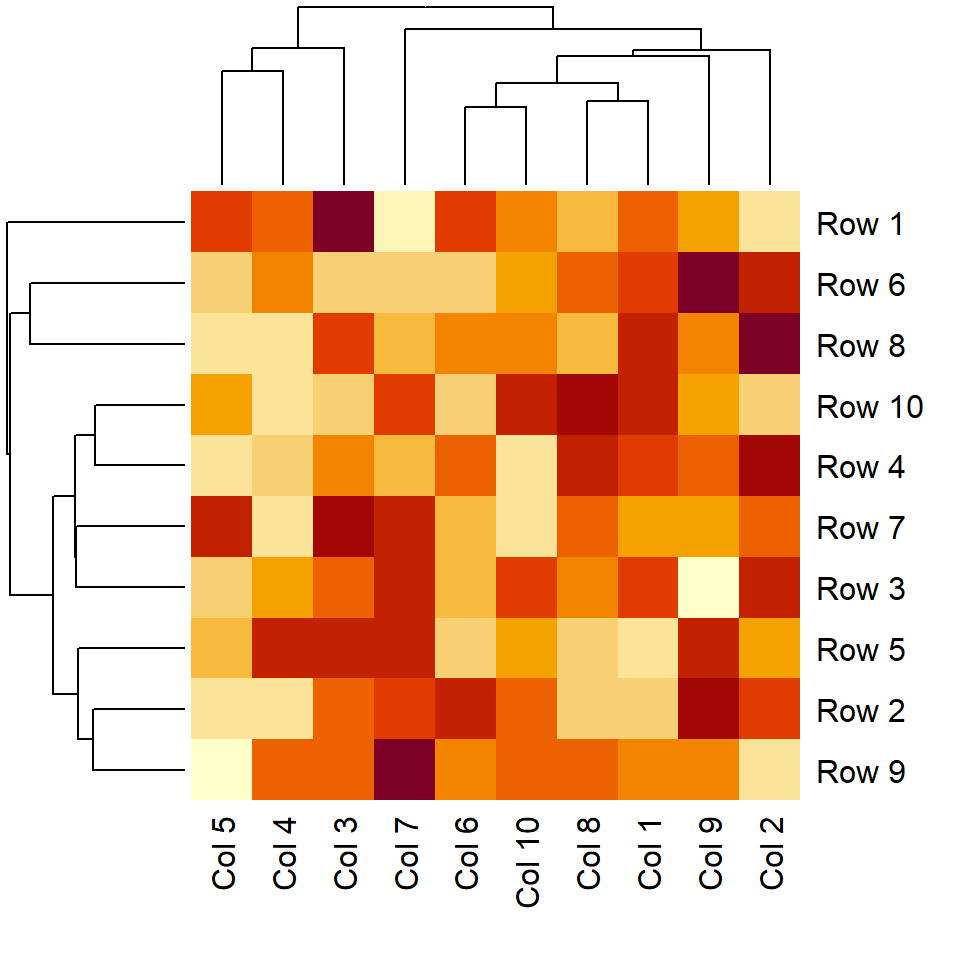Data normalization

The data is normalized by rows by default. However, you might need to normalize it in the column direction (`"column"`) or if no normalization must be carried at all (`"none"`).

``````# Matrix
m <- matrix(rnorm(100), ncol = 10)
colnames(m) <- paste("Col", 1:10)
rownames(m) <- paste("Row", 1:10)

heatmap(m, scale = "column")``````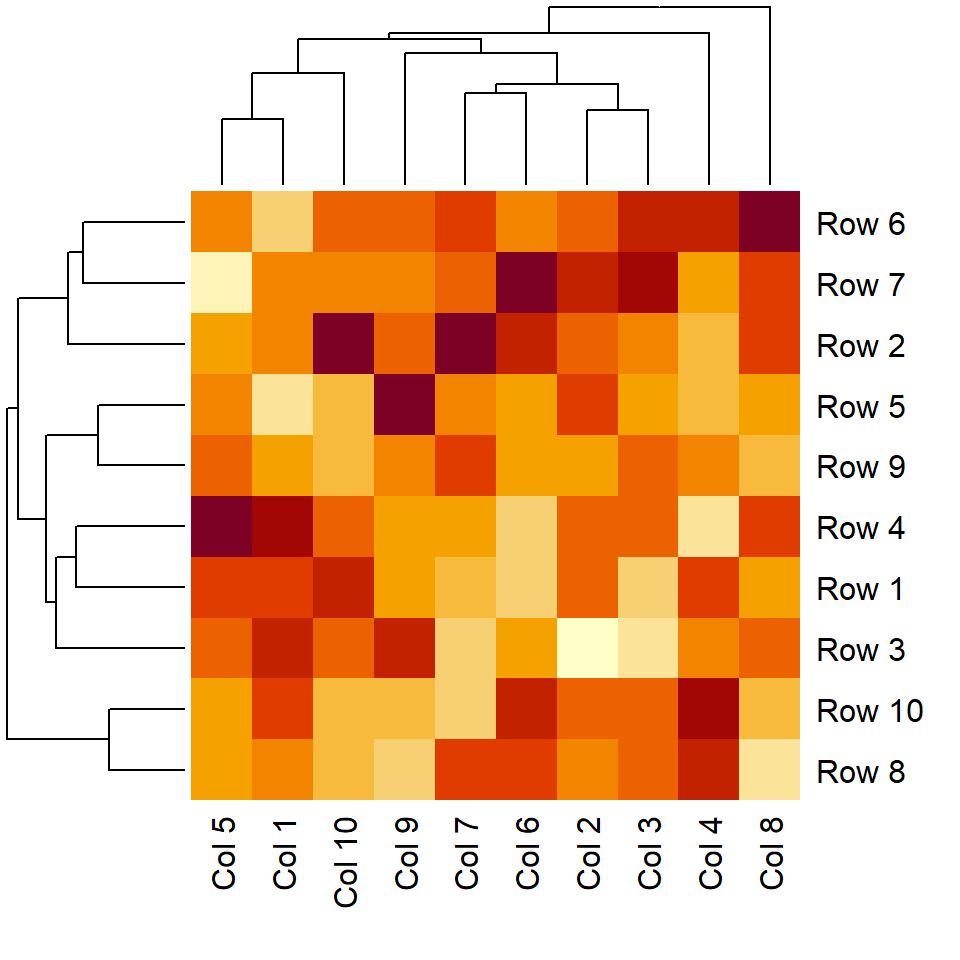Color customization

You can pass a color palette to the `col` argument of the `heatmap` function. In the following example we are using the viridis palette via `hcl.colors`.

``````# Matrix
m <- matrix(rnorm(100), ncol = 10)
colnames(m) <- paste("Col", 1:10)
rownames(m) <- paste("Row", 1:10)

heatmap(m, col = hcl.colors(50))``````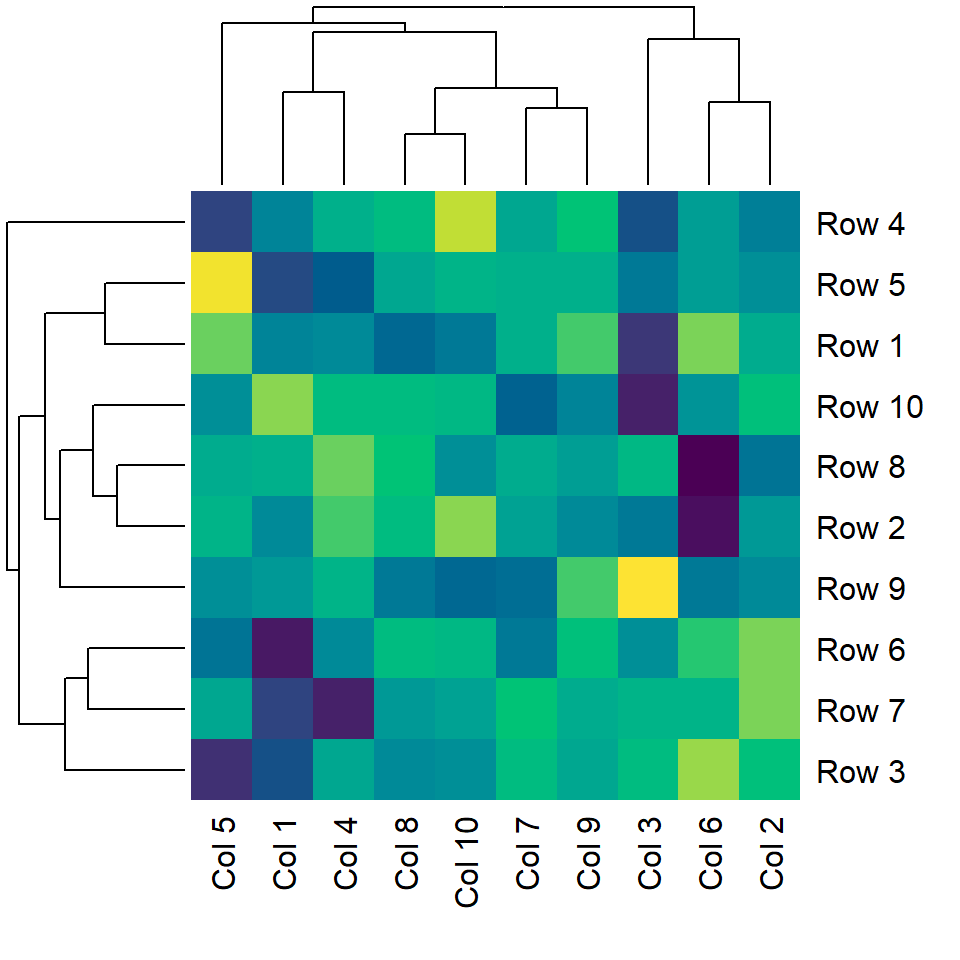Side colors

It is possible to pass a vector of colors to the `ColSideColors` and `RowSideColors` argument to annotate the columns and the rows of the matrix, respectively.

``````# Matrix
m <- matrix(rnorm(100), ncol = 10)
colnames(m) <- paste("Col", 1:10)
rownames(m) <- paste("Row", 1:10)

heatmap(m,
ColSideColors = rainbow(ncol(m)),
RowSideColors = rainbow(nrow(m)))``````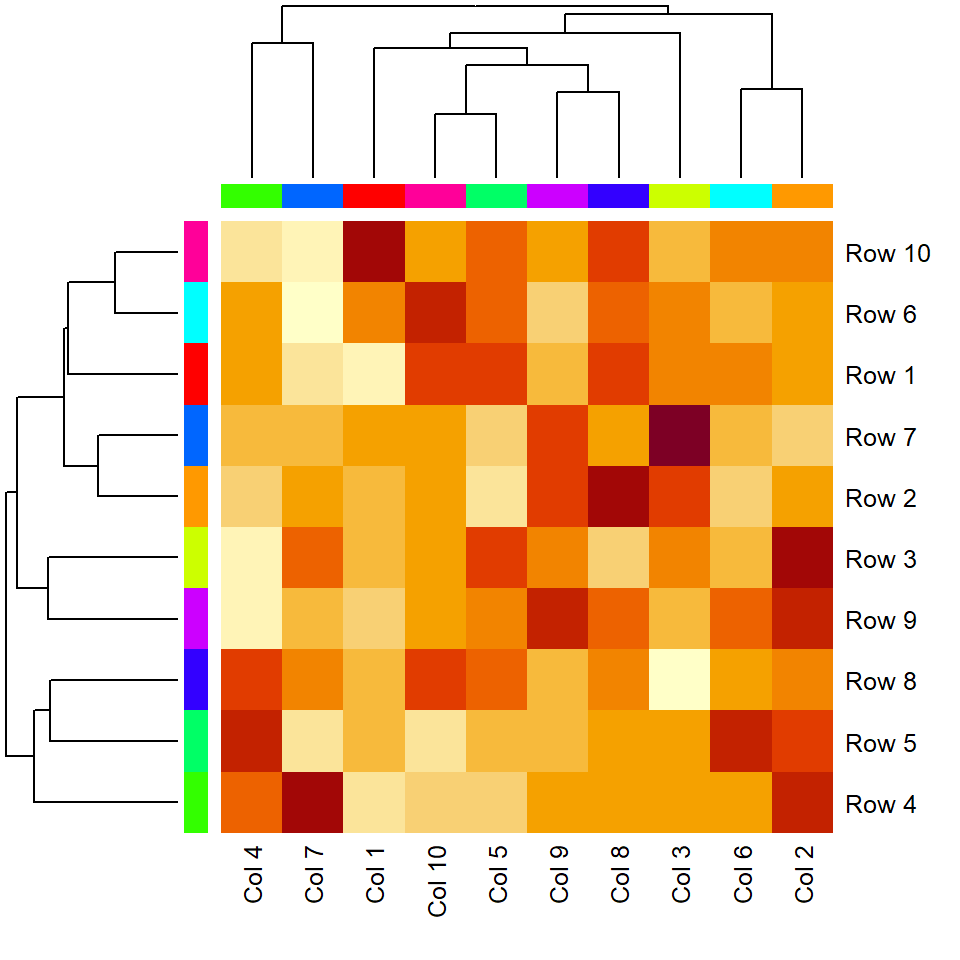## Heat map dendrograms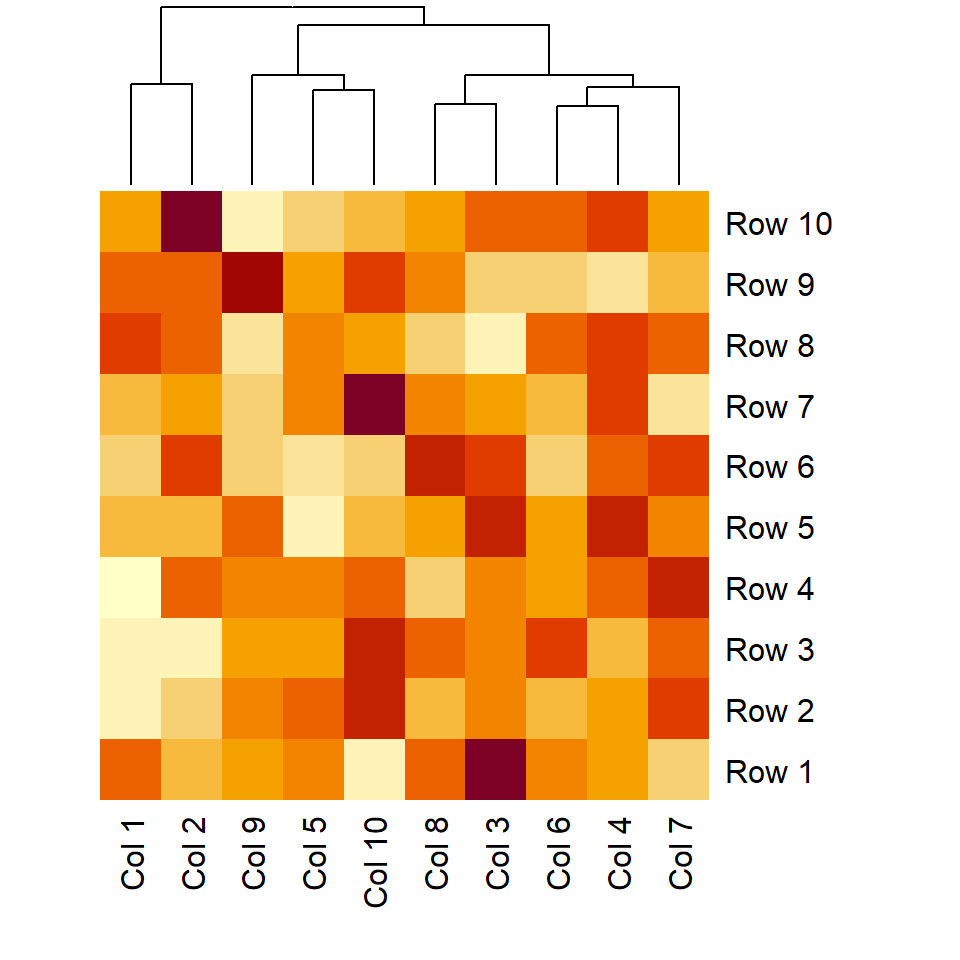Remove row dendrogram

The `Rowv` argument controls if the row dendrogram should be computed and how. You can pass a `dendrogram` or a vector specifying the order. Setting it to `NA` will remove the dendrogram.

``````# Matrix
m <- matrix(rnorm(100), ncol = 10)
colnames(m) <- paste("Col", 1:10)
rownames(m) <- paste("Row", 1:10)

heatmap(m, Rowv = NA)``````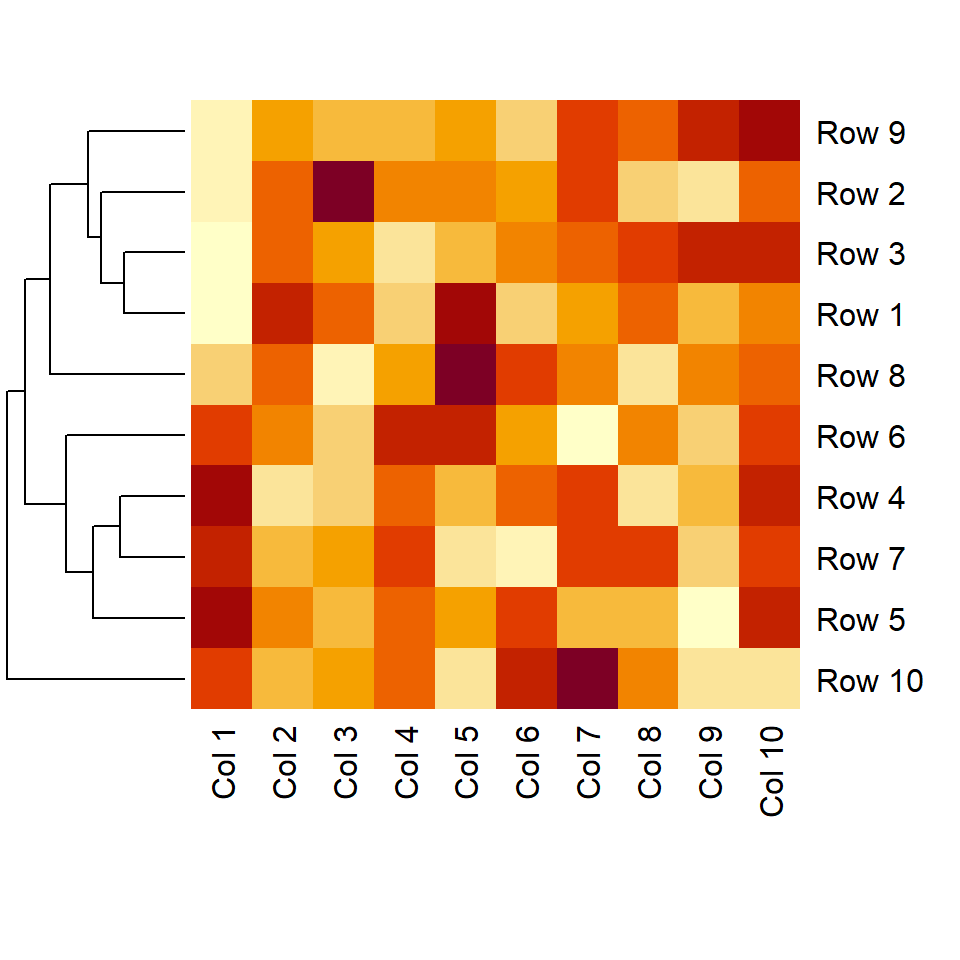Remove column dendrogram

The `Colv` argument is the equivalent of `Rowv` but for columns. Set it to `NA` to remove the column dendrogram.

``````# Matrix
m <- matrix(rnorm(100), ncol = 10)
colnames(m) <- paste("Col", 1:10)
rownames(m) <- paste("Row", 1:10)

heatmap(m, Colv = NA)``````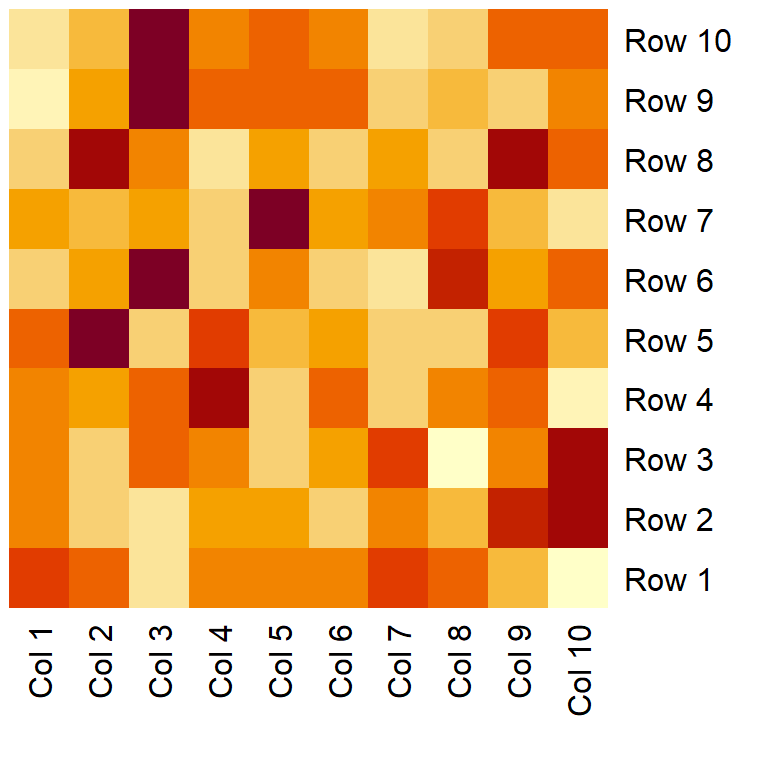Remove both dendrograms

Setting `Rowv` and `Colv` to `NA` will remove both dendrograms of the heat map and no reordering will be computed.

``````# Matrix
m <- matrix(rnorm(100), ncol = 10)
colnames(m) <- paste("Col", 1:10)
rownames(m) <- paste("Row", 1:10)

heatmap(m, Rowv = NA, Colv = NA)``````